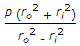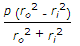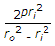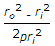# Mechanical Engineering - Strength of Materials

### Exercise :: Strength of Materials - Section 1

6.

A steel bar of 5 mm is heated from 15° C to 40° C and it is free to expand. The bar Will induce

 A. no stress B. shear stress C. tensile stress D. compressive stress

Explanation:

No answer description available for this question. Let us discuss.

7.

A body is subjected to a tensile stress of 1200 MPa on one plane and another tensile stress of 600 MPa on a plane at right angles to the former. It is also subjected to a shear stress of 400 MPa on the same planes. The maximum normal stress will be

 A. 400 MPa B. 500 MPa C. 900 MPa D. 1400 MPa

Explanation:

No answer description available for this question. Let us discuss.

8.

Two shafts 'A' and 'B' transmit the same power. The speed of shaft 'A' is 250 r.p.m. and that of shaft 'B' is 300 r.p.m. The shaft 'B' has the greater diameter.

 A. True B. False

Explanation:

No answer description available for this question. Let us discuss.

9.

A thick cylindrical shell having ro and ri as outer and inner radii, is subjected to an internal pressure (p). The maximum tangential stress at the inner surface of the shell is

 A.B.C.D.Explanation:

No answer description available for this question. Let us discuss.

10.

The stress induced in a body, when suddenly loaded, is __________ the stress induced when the same load is applied gradually.

 A. equal to B. one-half C. twice D. four times